##### Statistics II For Dummies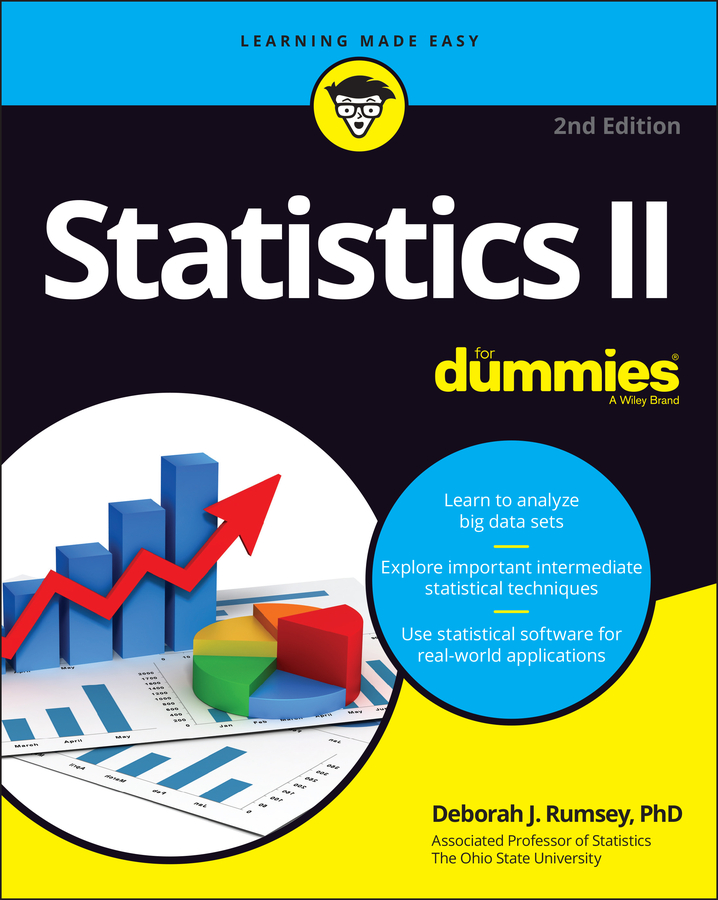The idea behind the limit comparison test is that if you take a known convergent series and multiply each of its terms by some number, then that new series also converges. And it doesn’t matter whether the multiplier is, say, 100, or 10,000, or 1/10,000 because any number, big or small, times the finite sum of the original series is still a finite number. The same thing goes for a divergent series multiplied by any number. That new series also diverges because any number, big or small, times infinity is still infinity. This is over simplified — it’s only in the limit that one series is sort of a multiple of the other — but it conveys the basic principle.

You can discover whether such a connection exists between two series by looking at the ratio of the nth terms of the two series as n approaches infinity. Here’s the test.

Limit Comparison Test: For two series,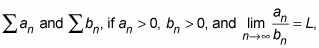where L is finite and positive, either both series converge or both diverge.

This is a good test to use when you can’t use the direct comparison test for your series because it goes the wrong way — in other words, your series is bigger than a known convergent series or smaller than a known divergent series.

Here’s an example: Does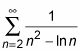converge or diverge? This series resembles the convergent p-series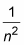so that’s your benchmark. But you can’t use the direct comparison test because the terms of your series are bigger than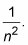Instead, you use the limit comparison test.

Take the limit of the ratio of the nth terms of the two series. It doesn’t matter which series you put in the numerator and which in the denominator, but if you put the known, benchmark series in the denominator, this makes it a little easier to do these problems and to grasp the results.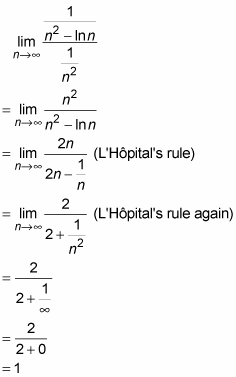Because the limit is finite and positive, and because the benchmark series converges, your series must also converge.

Thus,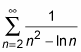converges.

Let’s try another example. Determine the convergence or divergence of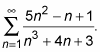The limit comparison test is a good one for series, like this one, in which the general term is a rational function — in other words, where the general term is a quotient of two polynomials.

1. Determine the benchmark series.

Take the highest power of n in the numerator and the denominator — ignoring any coefficients and all other terms — then simplify. Like this: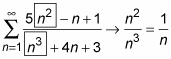The benchmark series is thus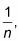the divergent harmonic series.

2. Take the limit of the ratio of the nth terms of the two series.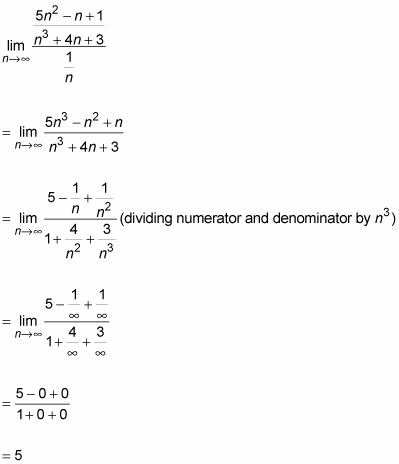3. Because the limit from Step 2 is finite and positive, and because the benchmark series diverges, your series must also diverge.

Thus,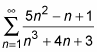diverges.

Contrary to the formal definition of the Limit Comparison Test (at the beginning of this article), the limit, L, doesn’t have to be finite and positive for the test to work. First, if the benchmark series is convergent, and you put it in the denominator of the limit, and the limit is zero, then your series must also converge. If the limit is infinity, you can’t conclude anything. And second, if the benchmark series is divergent, and you put it in the denominator, and the limit is infinity, then your series must also diverge. If the limit is zero, you learn nothing.